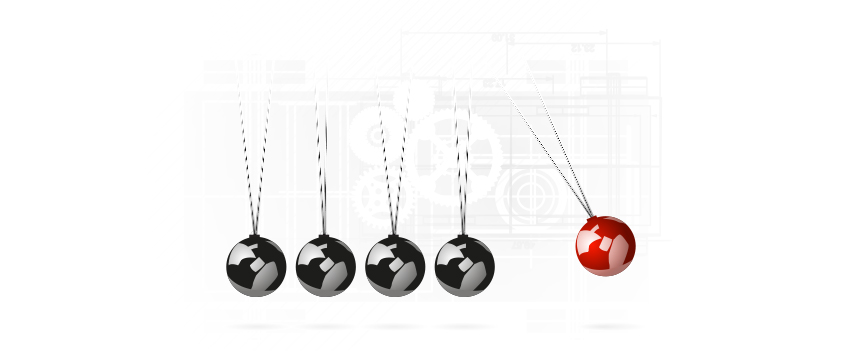# Pascal (Unit)

Units

The pascal (symbol: Pa) is the SI derived unit of pressure, internal pressure, stress, Young s modules and tensile strength, defined as one newton per square metre. Pressure is a measure of force per unit area. It is named after the French mathematician, physicist, inventor, writer, and philosopher Blaise Pascal.

Common multiple units of the pascal are the hectopascal (1 hPa ≡ 100 Pa) which is equal to 1 mbar, the kilopascal (1 kPa ≡ 1000 Pa), the megapascal (1 MPa ≡ 1,000,000 Pa), and the gigapascal (1 GPa ≡ 1,000,000,000 Pa).Rheology and petrology work with much higher pressures. That is why we do include:

• kilopascal (symbol kPa, thousand pascal) or 0,01 bar
• megapascal (symbol MPa, 1 million pascal) or 10 bar
• gigapascal (symbol GPa, 1 million pascal) or 10 000 bar.

The opposite occurs in vacuum technology, where pressures are lower than atmospheric:

• 1 pascal = 0,01 millibar
Prefix
Factor Name Symbol
100 pascal Pa
102 hectopascal hPa
103 kilopascal kPa
106 megapascal MPa
109 gigapascal GPa

#### Eenheden

Nieuwsgierig geworden?

Bel of mail ons.